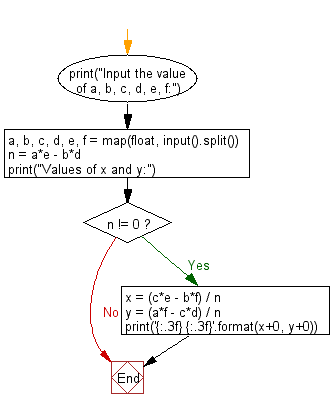﻿ Python: Solve the specified equation - w3resource# Python: Solve the specified equation

## Python Basic - 1: Exercise-35 with Solution

Write a Python program which solve the equation:
ax+by=c
dx+ey=f
Print the values of x, y where a, b, c, d, e and f are given.

Input:
a,b,c,d,e,f separated by a single space.
(-1,000 ≤ a,b,c,d,e,f ≤ 1,000)
Input the value of a, b, c, d, e, f:
5 8 6 7 9 4
Values of x and y:
-2.000 2.000

Sample Solution:

Python Code:

``````print("Input the value of a, b, c, d, e, f:")
a, b, c, d, e, f = map(float, input().split())
n = a*e - b*d
print("Values of x and y:")
if n != 0:
x = (c*e - b*f) / n
y = (a*f - c*d) / n
print('{:.3f} {:.3f}'.format(x+0, y+0))
``````

Sample Output:

```Input the value of a, b, c, d, e, f:
5 8 6 7 9 4
Values of x and y:
-2.000 2.000
```

Flowchart:Python Code Editor:

Have another way to solve this solution? Contribute your code (and comments) through Disqus.

What is the difficulty level of this exercise?

Test your Programming skills with w3resource's quiz.

﻿

## Python: Tips of the Day

What is the difference between Python's list methods append and extend?

append: Appends object at the end.

```x = [1, 2, 3]
x.append([4, 5])
print (x)
```

Output:

```[1, 2, 3, [4, 5]]
```

extend: Extends list by appending elements from the iterable.

```x = [1, 2, 3]
x.extend([4, 5])
print (x)
```

Output:

```[1, 2, 3, 4, 5]
```

Ref: https://bit.ly/2AZ6ZFq